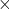## Problem 7.17 A horizontal spring with stiffness 0.8 N/m has a relaxed length of 12 cm (0.12 m). A mass of 23 grams (0.023 kg) is attached an

Question

Problem 7.17 A horizontal spring with stiffness 0.8 N/m has a relaxed length of 12 cm (0.12 m). A mass of 23 grams (0.023 kg) is attached and you stretch the spring to a total length of 27 cm (0.27 m). The mass is then released from rest. What is the speed of the mass at the moment when the spring returns to its relaxed length of 12 cm (0.12 m)?

in progress 0
5 months 2021-08-25T17:40:41+00:00 1 Answers 0 views 0

## Answers ( )

1. The speed of the mass v = 0.884 m/s.

Explanation:

Let

K1
represents the kinetic energy of the mass when it is released,

U1
represents the potential energy of the spring when the mass is released,

K2 represents the kinetic energy of the mass when the spring returns to relaxed length,

U2 represents the potential energy of the spring when the spring returns to relaxed length

The spring is stretched by 0.27 – 0.12 = 0.15 m

K1 = 0

U1 = (1/2)0.8(0.15)^2

= 0.009 J

U2 = 0

By conservation of energy,

K2 + U2 = K1 + U1

K2 + 0 = 0 + 0.009 J

K2 = 0.009 J

Let v = speed of the mass

K2 = 1/2mv^2

m = 23 g = 0.023 kg

0.009 = 1/20.023v^2

0.009 = 0.0115v^2

v = √(0.009 / 0.0115)

v = 0.884 m/s.